# Oops...

This website is made for modern browsers. You are seeing this, because your browser is missing a feature or two. Please install the latest update or switch to a modern browser. See you soon.

# Generate slices from a 3d model

In this tutorial, we will generate slices from a 3d model and arrange them next to each other in one layer. This approach will work with any three-dimensional object; here we use a cone. The slices result from an intersection of the model with an array of planes. The generated section curves could be used for plotting or a CNC router.

## Grasshopper

#### 1

##### Define initial geometric object

To start, we need a shape and in the following we will use a cone to illustrate the procedure. Thus, we place a Cone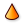Cone (Cone)
Surface  >  Primitive  >  Cone
Create a conical surface
Inputs
Base (B)Base plane
Length (L)Cone height
Outputs
Cone (C)Resulting cone
Tip (T)Tip of cone
on the canvas and assign the value of 10 to the radius R and length L. By attaching an Extrude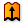Extrude (Extr)
Surface  >  Freeform  >  Extrude
Extrude curves and surfaces along a vector.
Inputs
Base (B)Profile curve or surface
Direction (D)Extrusion direction
Outputs
Extrusion (E)Extrusion result
component, we can turn the surface into a volumetric Brep. The direction D of the extrusion is given by a Unit Z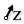Unit Z (Z)
Vector  >  Vector  >  Unit Z
Unit vector parallel to the world {z} axis.
Inputs
Factor (F)Unit multiplication
Outputs
Unit vector (V)World {z} vector
vector and a material thickness of -1. A Bounding BoxBounding Box (BBox)
Surface  >  Primitive  >  Bounding Box
Solve oriented geometry bounding boxes.
Inputs
Content (C)Geometry to contain
Plane (P)BoundingBox orientation plane
Outputs
Box (B)Aligned bounding box in world coordinates
Box (B)Bounding box in orientation plane coordinates
will then create a box around our cone.
By default, the Bounding BoxBounding Box (BBox)
Surface  >  Primitive  >  Bounding Box
Solve oriented geometry bounding boxes.
Inputs
Content (C)Geometry to contain
Plane (P)BoundingBox orientation plane
Outputs
Box (B)Aligned bounding box in world coordinates
Box (B)Bounding box in orientation plane coordinates
component will create a box for each object (as indicated by the label). If there are more objects, the component needs to be right-clicked and the option Union Box selected. This will wrap all objects in a single box.
By default, the Bounding BoxBounding Box (BBox)
Surface  >  Primitive  >  Bounding Box
Solve oriented geometry bounding boxes.
Inputs
Content (C)Geometry to contain
Plane (P)BoundingBox orientation plane
Outputs
Box (B)Aligned bounding box in world coordinates
Box (B)Bounding box in orientation plane coordinates
component will create a box for each object (as indicated by the label). If there are more objects, the component needs to be right-clicked and the option Union Box selected. This will wrap all objects in a single box.
To change the orientation of the bounding box (and so the direction of the slices), we could provide a plane at input P.

#### 2

##### Generate section planes

To define section planes, we need an array of points that serve as origins for the planes. To find these points, we use Evaluate Box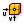Evaluate Box (Box)
Surface  >  Analysis  >  Evaluate Box
Evaluate a box in normalised {UVW} space.
Inputs
Box (B)Base box
U parameter (U){u} parameter (values between 0.0 and 1.0 are inside the box)
V parameter (V){v} parameter (values between 0.0 and 1.0 are inside the box)
W parameter (W){w} parameter (values between 0.0 and 1.0 are inside the box)
Outputs
Plane (Pl)Plane at {uvw} coordinate
Point (Pt)Point at {uvw} coordinate
Include (I)True if point is inside or on box
and can then attach parameters to U and V. The parameters have to be normalized (between 0 and 1).

The component Range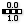Range (Range)
Sets  >  Sequence  >  Range
Create a range of numbers.
Inputs
Domain (D)Domain of numeric range
Steps (N)Number of steps
Outputs
Range (R)Range of numbers
will generate N values within the domain D. By default, the domain is 0 To 1 and 10 ascending values are created. This is good enough for this exercise, but we need to use Cull Index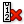Cull Index (Cull i)
Sets  >  Sequence  >  Cull Index
Cull (remove) indexed elements from a list.
Inputs
List (L)List to cull
Indices (I)Culling indices
Wrap (W)Wrap indices to list range
Outputs
List (L)Culled list
to remove the first (0) and last value (-1), which are the faces of the box. Then we connect the output of Range to the inputs U and V of Evaluate Box. This will give us the desired origin points at output Pt and we can connect a YZ Plane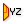YZ Plane (YZ)
Vector  >  Plane  >  YZ Plane
World YZ plane.
Inputs
Origin (O)Origin of plane
Outputs
Plane (P)World YZ plane
and an XZ Plane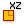XZ Plane (XZ)
Vector  >  Plane  >  XZ Plane
World XZ plane.
Inputs
Origin (O)Origin of plane
Outputs
Plane (P)World XZ plane
to get section planes in each direction.

#### 3

##### Compute section curves

While working with a Grasshopper algorithm, we have to ensure the data structures match the planed operation. In this case, we need a data tree

and use Entwine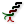Entwine (Entwine)
Sets  >  Tree  >  Entwine
Flatten and combine a collection of data streams
Inputs
Branch {0;0} ({0;0})Data to entwine
Branch {0;1} ({0;1})Data to entwine
Branch {0;2} ({0;2})Data to entwine
Outputs
Result (R)Entwined result
to forward both lists of planes. Each list will become separate branches on a single tree. The ZUI can be used to remove the unneeded input grip.

Next, we place a Brep | Plane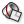Brep | Plane (Sec)
Intersect  >  Mathematical  >  Brep | Plane
Solve intersection events for a Brep and a plane (otherwise known as section).
Inputs
Brep (B)Base Brep
Plane (P)Section plane
Outputs
Curves (C)Section curves
Points (P)Section points
component on the canvas and connect our section planes and our solid (extruded cone) to it. At output C we find the curves that are created by solving the intersection event. But, each curve is in a separate branch and we need Trim TreeTrim Tree (Trim)
Sets  >  Tree  >  Trim Tree
Reduce the complexity of a tree by merging the outermost branches.
Inputs
Tree (T)Data tree to flatten
Depth (D)Number of outermost branches to merge
Outputs
Tree (T)Trimmed data tree
to remove the last separation level. Now we have two branches again; in each the curves for one direction. Optionally, we can bundle the curves in a Geometry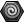Geometry (Geo)
Params  >  Geometry  >  Geometry
Contains a collection of generic geometry
container. The first task, slicing the model, is completed and we will now arrange the section curves on one layer.

#### 4

##### Get dimensions for arranging the curves in a plane

In the second half of this tutorial, we will arrange the slices in a single plane and next to each other, thus preparing them to be plotted on a sheet of paper. For this, we need coordinates for each slice that prevent overlapping of section curves. To get the dimensions, we use Plane Through Shape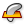Plane Through Shape (PxS)
Surface  >  Primitive  >  Plane Through Shape
Make a rectangular surface that is larger than a given shape.
Inputs
Plane (P)Surface plane
Shape (S)Shape to exceed
Inflate (I)Boundary inflation amount
Outputs
Surface (S)Resulting planar surface
, which creates a wrapping rectangle in plane P for each curve S.
The component Plane Through ShapePlane Through Shape (PxS)
Surface  >  Primitive  >  Plane Through Shape
Make a rectangular surface that is larger than a given shape.
Inputs
Plane (P)Surface plane
Shape (S)Shape to exceed
Inflate (I)Boundary inflation amount
Outputs
Surface (S)Resulting planar surface
is similar to Bounding BoxBounding Box (BBox)
Surface  >  Primitive  >  Bounding Box
Solve oriented geometry bounding boxes.
Inputs
Content (C)Geometry to contain
Plane (P)BoundingBox orientation plane
Outputs
Box (B)Aligned bounding box in world coordinates
Box (B)Bounding box in orientation plane coordinates
, but instead of a box with three dimensions, we get a planar, two-dimensional frame.
The component Plane Through ShapePlane Through Shape (PxS)
Surface  >  Primitive  >  Plane Through Shape
Make a rectangular surface that is larger than a given shape.
Inputs
Plane (P)Surface plane
Shape (S)Shape to exceed
Inflate (I)Boundary inflation amount
Outputs
Surface (S)Resulting planar surface
is similar to Bounding BoxBounding Box (BBox)
Surface  >  Primitive  >  Bounding Box
Solve oriented geometry bounding boxes.
Inputs
Content (C)Geometry to contain
Plane (P)BoundingBox orientation plane
Outputs
Box (B)Aligned bounding box in world coordinates
Box (B)Bounding box in orientation plane coordinates
, but instead of a box with three dimensions, we get a planar, two-dimensional frame.
The actual dimensions are calculated with the component Dimensions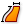Dimensions (Dim)
Surface  >  Analysis  >  Dimensions
Get the approximate dimensions of a surface
Inputs
Surface (S)Surface to measure
Outputs
U dimension (U)Approximate dimension in U direction
V dimension (V)Approximate dimension in V direction
.

#### 5

##### Find coordinates in x-direction

As stated, the coordinates for each slice on our base place depend on the dimensions of the section curves. Here, we would like to have two rows of section profiles, separated for each slicing direction. In x-direction we will place the curves from the same set and their distance is given by the height of each slice. The heights are obtained from output V of DimensionsDimensions (Dim)
Surface  >  Analysis  >  Dimensions
Get the approximate dimensions of a surface
Inputs
Surface (S)Surface to measure
Outputs
U dimension (U)Approximate dimension in U direction
V dimension (V)Approximate dimension in V direction
.

The list of x-coordinates ought to start at 0, but currently starts with the first height. Therefore, we use Shift List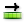Shift List (Shift)
Sets  >  List  >  Shift List
Offset all items in a list.
Inputs
List (L)List to shift
Shift (S)Shift offset
Wrap (W)Wrap values
Outputs
List (L)Shifted list
to offset the list by -1. Then we use Replace ItemsReplace Items (Replace)
Sets  >  List  >  Replace Items
Replace certain items in a list.
Inputs
List (L)List to modify
Item (I)Items to replace with. If no items are supplied, nulls will be inserted.
Indices (i)Replacement index for each item
Wrap (W)If true, indices will be wrapped
Outputs
List (L)List with replaced values
to replace the first item (index 0) in the list with 0.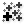Mass Addition (MA)
Maths  >  Operators  >  Mass Addition
Perform mass addition of a list of items
Inputs
Input (I)Input values for mass addition.
Outputs
Partial Results (Pr)List of partial results
sums all values in a list and also returns the intermediate results at output Pr. This is what we will use for the x-coordinates.

#### 6

##### Find coordinates in y-direction

For the coordinates in y-direction, we will use a different approach, because here we will just have two rows, one for each set of curves. The distance of the rows is given by the largest width in the first set. The widths can be found at output U of DimensionsDimensions (Dim)
Surface  >  Analysis  >  Dimensions
Get the approximate dimensions of a surface
Inputs
Surface (S)Surface to measure
Outputs
U dimension (U)Approximate dimension in U direction
V dimension (V)Approximate dimension in V direction
and we use Explode Tree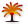Explode Tree (BANG!)
Sets  >  Tree  >  Explode Tree
Extract all the branches from a tree
Inputs
Data (D)Data to explode
Outputs
Branch 0 (-)All data inside the branch at index: 0
Branch 1 (-)All data inside the branch at index: 1
to separate the widths for each slicing direction. We are interested in the widths of the first branch {0,0}, which is also the first row of section curves.

To find the largest width, we use Sort ListSort List (Sort)
Sets  >  List  >  Sort List
Sort a list of numeric keys.
Inputs
Keys (K)List of sortable keys
Values A (A)Optional list of values to sort synchronously
Outputs
Keys (K)Sorted keys
Values A (A)Synchronous values in A
to get an ascending order. Then, a List Item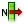List Item (Item)
Sets  >  List  >  List Item
Retrieve a specific item from a list.
Inputs
List (L)Base list
Index (i)Item index
Wrap (W)Wrap index to list bounds
Outputs
Item (i)Item at {i'}
component will get the first value and thus we Reverse its input L to find the largest value. This will become the y-coordinate for the second row. The first row starts at 0 and we use EntwineEntwine (Entwine)
Sets  >  Tree  >  Entwine
Flatten and combine a collection of data streams
Inputs
Branch {0;0} ({0;0})Data to entwine
Branch {0;1} ({0;1})Data to entwine
Branch {0;2} ({0;2})Data to entwine
Outputs
Result (R)Entwined result
to create two branches with one y-coordinate in each.

#### 7

##### Arrange slices in a plane

After calculating the coordinates for each slice, we arrange them all in a single plane. To convert the coordinates to actual points, we will use Construct Point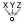Construct Point (Pt)
Vector  >  Point  >  Construct Point
Construct a point from {xyz} coordinates.
Inputs
X coordinate (X){x} coordinate
Y coordinate (Y){y} coordinate
Z coordinate (Z){z} coordinate
Outputs
Point (Pt)Point coordinate
and connect the inputs X and Y with the results from the previous two steps.

To place the section curves at their coordinates, we use Orient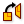Orient (Orient)
Transform  >  Euclidean  >  Orient
Orient an object. Orientation is sometimes called a 'ChangeBasis tranformation'. It allows for remapping of geometry from one axis-system to another.
Inputs
Geometry (G)Base geometry
Source (A)Initial plane
Target (B)Final plane
Outputs
Geometry (G)Reoriented geometry
Transform (X)Transformation data
: Input G takes the geometric objects (our curves), input A the reference planes (result of Plane Through Shape) and input B the target planes (generated by connecting our coordinates).

The result of this tutorial could now be plotted or fabricated with a CNC machine

. It’s also possible to create slots at the slices' intersections to create a sliceform, which is explained in the tutorial Create a sliceform.

## Get the results

### Version Info

• Rhino 6.31
• Grasshopper 1.0.0007

In the tutorial above, there was one geometric object to be sliced. Usually, we have more than one and now it’s your turn to tweak the algorithm so that it can handle several geometric objects. The section profiles on the base plane should contain all curves from one section. To keep it simple, let’s add a sphere to the tip of the cone.

Hint 1

### Create the sphere

First, we create the sphere with Sphere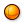Sphere (Sph)
Surface  >  Primitive  >  Sphere
Create a spherical surface.
Inputs
Base (B)Base plane
Outputs
Sphere (S)Resulting sphere
and set a radius. Now, the sphere must become part of the algorithm.

Hint 2

### Connect the sphere

To include the sphere, we connect it to input C of Bounding BoxBounding Box (BBox)
Surface  >  Primitive  >  Bounding Box
Solve oriented geometry bounding boxes.
Inputs
Content (C)Geometry to contain
Plane (P)BoundingBox orientation plane
Outputs
Box (B)Aligned bounding box in world coordinates
Box (B)Bounding box in orientation plane coordinates
and to input B of the component Brep | PlaneBrep | Plane (Sec)
Intersect  >  Mathematical  >  Brep | Plane
Solve intersection events for a Brep and a plane (otherwise known as section).
Inputs
Brep (B)Base Brep
Plane (P)Section plane
Outputs
Curves (C)Section curves
Points (P)Section points
. But, this does not yet lead to the desired result and we need to change the handling of data in the algorithm.

Hint 3

### Fix data structure

To change the data handling, we right-click the component Bounding BoxBounding Box (BBox)
Surface  >  Primitive  >  Bounding Box
Solve oriented geometry bounding boxes.
Inputs
Content (C)Geometry to contain
Plane (P)BoundingBox orientation plane
Outputs
Box (B)Aligned bounding box in world coordinates
Box (B)Bounding box in orientation plane coordinates
and select the option Union Box. This will create one box around all objects and not wrap each object with its own box.

Also, we have graft input P of Brep | PlaneBrep | Plane (Sec)
Intersect  >  Mathematical  >  Brep | Plane
Solve intersection events for a Brep and a plane (otherwise known as section).
Inputs
Brep (B)Base Brep
Plane (P)Section plane
Outputs
Curves (C)Section curves
Points (P)Section points
. Now, every section plane is in its own branch and every item at input B will be matched with every plane. This change will lead to an error: the component is displayed in red and states “Intersection failed”. But, this result is correct and could have been expected; the radius of the sphere is not large enough for it to have an intersection event with all the planes. We can ignore this error.

In the Rhino viewport, we can see that the arrangement on one layer is not yet working as expected. This is caused by the changed data structure; Plane Through ShapePlane Through Shape (PxS)
Surface  >  Primitive  >  Plane Through Shape
Make a rectangular surface that is larger than a given shape.
Inputs
Plane (P)Surface plane
Shape (S)Shape to exceed
Inflate (I)Boundary inflation amount
Outputs
Surface (S)Resulting planar surface
needs our attention.

Hint 4

### Capture the items in a single plane

The problem is that Plane Through ShapePlane Through Shape (PxS)
Surface  >  Primitive  >  Plane Through Shape
Make a rectangular surface that is larger than a given shape.
Inputs
Plane (P)Surface plane
Shape (S)Shape to exceed
Inflate (I)Boundary inflation amount
Outputs
Surface (S)Resulting planar surface
is getting two items in each list, after solving the intersection with Brep | PlaneBrep | Plane (Sec)
Intersect  >  Mathematical  >  Brep | Plane
Solve intersection events for a Brep and a plane (otherwise known as section).
Inputs
Brep (B)Base Brep
Plane (P)Section plane
Outputs
Curves (C)Section curves
Points (P)Section points
and Trim TreeTrim Tree (Trim)
Sets  >  Tree  >  Trim Tree
Reduce the complexity of a tree by merging the outermost branches.
Inputs
Tree (T)Data tree to flatten
Depth (D)Number of outermost branches to merge
Outputs
Tree (T)Trimmed data tree
. Thus, Plane Through Shape creates a bounding rectangle for each item, but this is not what we want. Instead, we need a bounding rectangle both around items.

The Plane Through Shape component has no option for this and we use another Bounding BoxBounding Box (BBox)
Surface  >  Primitive  >  Bounding Box
Solve oriented geometry bounding boxes.
Inputs
Content (C)Geometry to contain
Plane (P)BoundingBox orientation plane
Outputs
Box (B)Aligned bounding box in world coordinates
Box (B)Bounding box in orientation plane coordinates
with option Union Box selected. This way, we get flat boxes with no height. We have to reduce them by a branch level with Trim TreeTrim Tree (Trim)
Sets  >  Tree  >  Trim Tree
Reduce the complexity of a tree by merging the outermost branches.
Inputs
Tree (T)Data tree to flatten
Depth (D)Number of outermost branches to merge
Outputs
Tree (T)Trimmed data tree
and can now connect them to Plane Through ShapePlane Through Shape (PxS)
Surface  >  Primitive  >  Plane Through Shape
Make a rectangular surface that is larger than a given shape.
Inputs
Plane (P)Surface plane
Shape (S)Shape to exceed
Inflate (I)Boundary inflation amount
Outputs
Surface (S)Resulting planar surface
to find the rectangle around all intersection curves in a slice.
Instead of connecting the results from Bounding Box to Plane Through Shape, which is kind of an unnecessary repetition, we could have also extracted the right face from the box. But, more component would be needed.
Instead of connecting the results from Bounding Box to Plane Through Shape, which is kind of an unnecessary repetition, we could have also extracted the right face from the box. But, more component would be needed.

As we can see, we still need to adjust OrientOrient (Orient)
Transform  >  Euclidean  >  Orient
Orient an object. Orientation is sometimes called a ‘ChangeBasis tranformation’. It allows for remapping of geometry from one axis-system to another.
Inputs
Geometry (G)Base geometry
Source (A)Initial plane
Target (B)Final plane
Outputs
Geometry (G)Reoriented geometry
Transform (X)Transformation data
to handle the new data structure, which is done by grafing inputs A and B. Done.

Get the results

## Get the results

### Version Info

• Rhino 6.31
• Grasshopper 1.0.0007